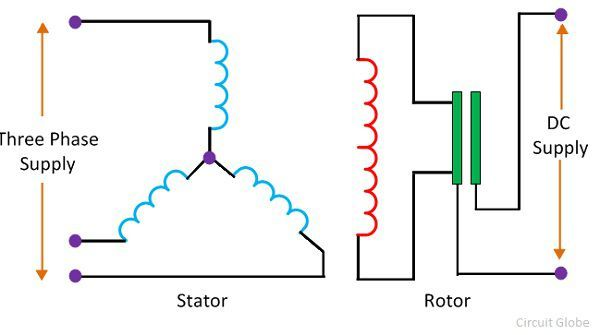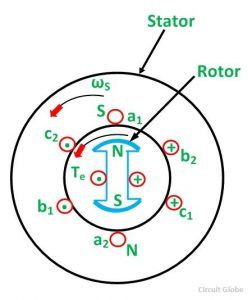# Working Principle of a Synchronous Motor

The stator and the rotor are the two main parts of the synchronous motor. The stator is the stationary part of the motor and rotor is their rotating part. The stator is excited by the three-phase supply, and the rotor is excited by the DC supply.

The term excitation means the magnetic field induces in the stator and rotor of the motor. The main aim of the excitation is to convert the stator and rotor into an electromagnet.The three-phase supply induces the north and south pole on the stator. The three-phase supply is sinusoidal. The polarity (positive and negative) of their wave changes after every half cycle and because of this reason the north and south pole also varies. Thus, we can say that the rotating magnetic field develops on the stator.

The magnetic field develops on the rotor because of the DC supply. The polarity of the DC supply becomes fixed, and thus the stationary magnetic field develops on the rotor.  The term stationary means their north and south pole remains fixed.

The speed at which the rotating magnetic field rotates is known as the synchronous speed. The synchronous speed of the motor depends on the frequency of the supply and the number of poles of the motor.

NS = 120f/P

When the opposite pole of the stator and rotor face each other, the force of attraction occurs between them. The attraction force develops the torque in the anti-clockwise direction. The torque is the kind of force which moves the object in the rotation. Thus, the poles of rotor dragged towards the poles of the stator.

After every half cycle, the pole on the stator is reversed. The position of the rotor remains same because of the inertia. The inertia is the tendency of an object to remain fixed in one position.

When the like pole of the stator and rotor face each other, the force of repulsion occurs between them and the torque develops in the clockwise direction.

Let understand this with the help of the diagram. For simplicity, consider the motor has two poles. In the below figure, the opposite pole of the stator and rotor face each other. So the attraction force develops between them.After the half cycle, the poles on the stator reverse. The same pole of the stator and rotor face each other, and the force of repulsion develops between them.The non-unidirectional torque pulsates the rotor only in one place and because of this reason the synchronous motor is not self-starting.For starting the motor, the rotor is rotated by some external means. Thus, the polarity of the rotor also changed along with the stator. The pole of the stator and rotor interlock each other and the unidirectional torque induces in the motor. The rotor starts rotating at the speed of the rotating magnetic field, or we can say at synchronous speed.

The speed of the motor is fixed, and the motor continuously rotates at the synchronous speed.